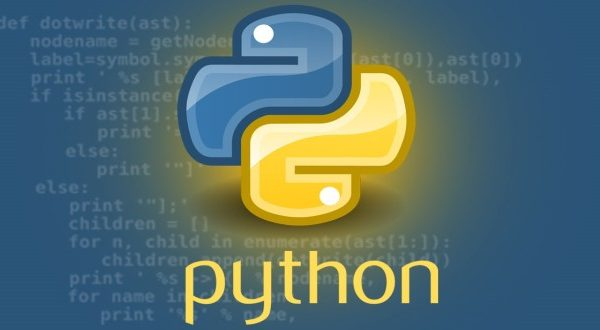# Multiple Linear Regression with Python### Introduction

Linear regression is one of the most commonly used algorithms in machine learning. You’ll want to get familiar with linear regression because you’ll need to use it if you’re trying to measure the relationship between two or more continuous values.

A deep dive into the theory and implementation of linear regression will help you understand this valuable machine learning algorithm.

### Defining Terms

Before we delve into linear regression, let’s take a moment to make sure we are clear on what regression is.

In machine learning, there are two different types of supervised learning methods: classification and regression.

In general, regression is a statistical method that estimates relationships between variables. Classification also attempts to find relationships between variables, with the main difference between classification and regression being the output of the model.

In a regression task, the output variable is numerical or continuous in nature, while for classification tasks the output variable is categorical or discrete in nature. If a variable is categorical it means that there is a finite/discrete number of groups or categories the variable can fit into.

Consider a classifier that tries to predict what type of mammal an animal is,